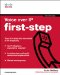# Chapter Summary

 This chapter explained how analog waveforms generated by the human voice are converted into digital data, which can then be sent across a network. Specifically, samples of an analog wave are taken at a rate of twice the highest frequency. For example, if the highest frequency being sampled is 4000 Hz, then 8000 samples (that is, 4000 * 2) are taken every second. This sampling is called (PAM. However, PAM does not digitize the signal. So, the next step is to assign numeric values to each of the PAM samples, based on the samples' volume (that is, amplitude). Each of these values can be represented numerically using 8 bits. Therefore, voice often requires 64,000 bits per second (that is, 4000 * 2 * 8) of bandwidth, not including additional overhead. This process is called PCM, which is how the G.711 CODEC operates. To conserve bandwidth, especially over relatively slow-speed WAN links, VoIP network designers often use a CODEC other than G.711 in order to compress the voice samples and to reduce bandwidth demands. In the Cisco VoIP environment, the G.729 CODEC, which only requires 8 kbps of bandwidth (not including overhead), is commonly used. Calculating the total bandwidth (including overhead) required by a VoIP call can be done using the Cisco web-based Voice Bandwidth Calculator. Because voice compression does somewhat sacrifice voice quality in favor of reduced bandwidth, being able to measure and quantify voice quality is critical. This chapter described three voice quality measurements: MOS, PSQM, and PESQ. Finally, this chapter delved into traffic engineering, which calculates the total amount of bandwidth needed to support peak traffic loads in a VoIP network. However, we do not typically purchase enough bandwidth to handle all calls occurring during that peak usage time. Otherwise, we would pay for bandwidth that was only used for brief periods.Voice over IP First-Step
ISBN: 1587201569
EAN: 2147483647
Year: 2005
Pages: 138
Authors: Kevin Wallace

Similar book on Amazon

flylib.com © 2008-2017.
If you may any questions please contact us: flylib@qtcs.net